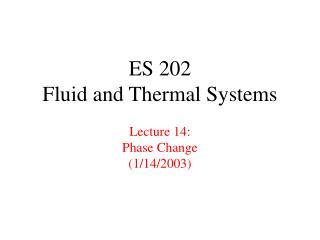DownloadDownload PresentationES 202 Fluid and Thermal Systems Lecture 14: Phase Change (1/14/2003)

# ES 202 Fluid and Thermal Systems Lecture 14: Phase Change (1/14/2003)

Télécharger la présentation## ES 202 Fluid and Thermal Systems Lecture 14: Phase Change (1/14/2003)

- - - - - - - - - - - - - - - - - - - - - - - - - - - E N D - - - - - - - - - - - - - - - - - - - - - - - - - - -
##### Presentation Transcript

1. ES 202Fluid and Thermal SystemsLecture 14:Phase Change(1/14/2003)

2. Assignments • Homework: • 3-2, 3-4, 3-6, 3-9 in Cengel & Turner • Reading assignment • Section 3-1 to 3-4, 3-6 in Cengel & Turner ES 202 Fluid & Thermal Systems

3. Announcements • A note on Lab 2 write-up • due date • one write-up per lab group (higher expectation) • Contents: water wall, Torricelli experiment, pipe friction • Advice: start early, discuss with me if you need advice • Homework policy for Problem 11-105 • not an easy problem • contains many concepts in a complete solution • Goal of homework: facilitate your learning • Feedback on Exam 1 from you ES 202 Fluid & Thermal Systems

4. Road Map of Lecture 14 • Background quiz • Phase change • example: the boiling process • difference in molecular structure • State Principle • representation of P-v-T surface • two dimensional projection of P-v-T surface • terminology and definition • models: • ideal gas • incompressible substance ES 202 Fluid & Thermal Systems

5. Background Quiz • How many phases does a substance have? • solid • liquid • gas • Zeroth law of thermodynamics • Statement: If two bodies are in thermal equilibrium with a third body, they are also in thermal equilibrium with each other. • Significance: Introduce the concept of temperature • First law of thermodynamics • Significance: Conservation of energy • Second law of thermodynamics • Significance: Entropycannot be destroyed ES 202 Fluid & Thermal Systems

6. Background Quiz (Cont’d) • Energy balance for an open system: • Entropy balance for an open system: ES 202 Fluid & Thermal Systems

7. Background Quiz (Cont’d) • Simple substance: • there is only one reversible work mode which can change the energy of the system • think of work mode as an independent control knob (apart from heat transfer) which can change the energy of a system • State Principle: • Two independent, intensive thermodynamic properties are sufficient to fully determine the thermodynamic state of a simple substance. • For example: • Example from ideal gas model: ES 202 Fluid & Thermal Systems

8. The Boiling Process • Exercise: Sketch the temperature variation of a pot of water from the moment that you turn on the stove • Question: The temperature does not change even though you keep adding heat to the system. Where does the energy go? • Question: What happens to the water during the boiling process, i.e. its internal structure? • Question: What is the surrounding pressure on the water? ES 202 Fluid & Thermal Systems

9. P-v-T Surface • State Principle: • Two independent, intensive thermodynamic properties are sufficient to fully determine the thermodynamic state of a simple substance. • One can visualize the thermodynamic state of a simple substance on a 3D surface • Show the P-v-T surface • identify the solid, liquid, vapor regions • identify the two-phase dome ES 202 Fluid & Thermal Systems

10. Two-Dimensional Representation • The 3D surface can be represented by multiple contour lines on a 2D plane, for example: • P-v plane (direction of increasing temperature) • T-v plane (direction of increasing pressure) • P-T plane • Sketch these above planes for: • an ideal gas • an incompressible substance • Where are the ideal gas model and incompressible substance state located on the P-v-T surface relative to the two-phase dome? ES 202 Fluid & Thermal Systems

11. Terminology and Definition • Pure substance • Compressed liquid • Saturated liquid • Saturated vapor • Superheated vapor • The two-phase (vapor) dome • Saturated liquid line • Saturated vapor line • Critical point • Triple point • Quality • Trace the boiling process on the T-v plane • effect of imposed pressure on boiling point ES 202 Fluid & Thermal Systems